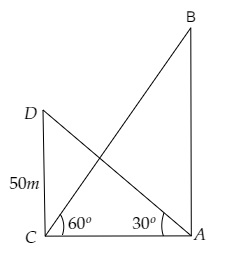# The angle of elevation of the top of a hill at the foot of a tower is $60^{\circ}$ and the angle of elevation of the top of the tower from the foot of the hill is $30^{\circ}$. If the tower is $50 \mathrm{~m}$ high, what is the height of the hill?

Given:

The angle of elevation of the top of a hill at the foot of a tower is $60^{\circ}$ and the angle of elevation of the top of the tower from the foot of the hill is $30^{\circ}$. The tower is $50 \mathrm{~m}$ high.

To do:

We have to find the height of the hill.

Solution:Let $AB$ be the height of the hill and $CD$ be the height of the tower.

From the figure,

$\mathrm{CD}=50 \mathrm{~m}, \angle \mathrm{BCA}=60^{\circ}, \angle \mathrm{DAC}=30^{\circ}$

Let the height of the hill be $\mathrm{AB}=h \mathrm{~m}$ and the distance between the tower and the hill be $\mathrm{CA}=x \mathrm{~m}$.

We know that,

$\tan \theta=\frac{\text { Opposite }}{\text { Adjacent }}$

$=\frac{\text { AB }}{CA}$

$\Rightarrow \tan 60^{\circ}=\frac{h}{x}$

$\Rightarrow \sqrt3=\frac{h}{x}$

$\Rightarrow x=\frac{h}{\sqrt3} \mathrm{~m}$.........(i)

Similarly,

$\tan \theta=\frac{\text { Opposite }}{\text { Adjacent }}$

$=\frac{\text { DC }}{AC}$

$\Rightarrow \tan 30^{\circ}=\frac{50}{x}$

$\Rightarrow \frac{1}{\sqrt3}=\frac{50}{\frac{h}{\sqrt3}}$              [From (i)]

$\Rightarrow \frac{1}{\sqrt3}\times\frac{h}{\sqrt3}=50 \mathrm{~m}$

$\Rightarrow h=50(3)=150 \mathrm{~m}$

Therefore, the height of the hill is $150 \mathrm{~m}$.